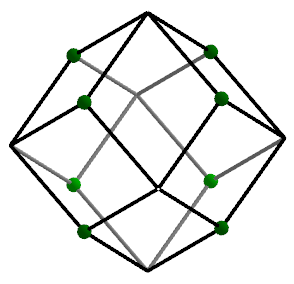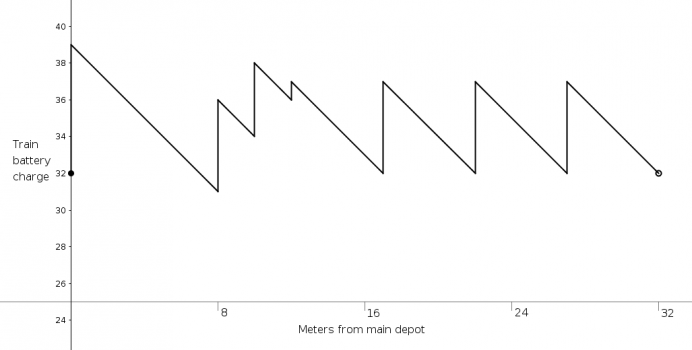Varsity Math, Week 52________________

With the new academic year underway, it’s time for the grueling tryouts for this year’s first string on the Varsity Math team. Here’s a sample of what the competitors are up against.

________________

Mean Triangle

You are given a right triangle with the property that the length of the median to the hypotenuse is the geometric mean (the square root of the product) of the lengths of the legs. The hypotenuse is ten units in length.

What is the length of the shorter leg?As Easy as 4132

Let’s call a sequence of four real numbers a “sumo sequence” if, whenever you add any one of them to the product of the other three, the sum is exactly two.

How many sumo sequences are there?

Solutions to week 51Rhombarium. There are many ways to attack this problem, but here’s one particularly simple one. Start by noticing that the vertices of the rhombic dodecahedron highlighted in the diagram at right lie at the vertices of a perfect cube. Moreover, if you imagine removing that cube from the rhombic dodecahedron, six identical square pyramids remain. Since each original rhombic face is planar, if you rotate any one of the square pyramids 180° around an edge of the (removed) cube, one triangular face of that pyramid exactly coincides with a triangular face of the adjacent pyramid, and the square faces end up at right angles to each other. Continuing with other square pyramids similarly, you can see that the six square pyramids precisely assemble into a cube identical to the removed one. Hence, the volume of the rhombarium is just twice the volume of that inscribed cube, whose edge is the short diagonal of the rhombic faces. With an edge length of 5√3 and a long diagonal √2 the length of the short diagonal, the Pythagorean theorem shows that the sides of the right triangle formed by two half-diagonals and one edge of a rhombus are 5, 5√2, and 5√3 cm, respectively. Hence, the short diagonal is 10cm, the removed cube has a volume of one liter, and the total volume of the rhombarium is two liters.

Well Trained. First, imagine starting at the main depot with 32 watt-hours (Wh) of charge before charging — enough so that the train will make it all the way around the track no matter what happens. Then the graph of the charge level in the train’s batteries as a function of distance traveled around the track, including the charging, is shown below. Note that the lowest battery charge occurs just before charging at the first station eight meters beyond the main depot. If the train started there with zero charge in its battery (which can be visualized just by mentally moving the x-axis up so it just touches the graph at this point) the train will have non-zero charge in its battery all the way around, and will come back exactly to this point with zero charge ready to charge up again at this station, since it both acquires and expends 32 Wh in making it around the track once. There is only one lowest point on the graph, so the unique place the train can start on an empty battery is 8 meters past the main depot.Coach Newton elaborates:
This argument actually shows something much more general. In any closed circuit, if the resources consumed matches the resources acquired in the execution of a single circuit, then there will be someplace in the circuit that the process can start with zero resources and yet complete the entire circuit without running out of resources. This could be a vehicle with fuel, or a carnivalgoer with tickets, or any setting in which some resource is both acquired and consumed in various places on a closed path.

Recent Weeks

Links to all of the puzzles and solutions are on the Complete Varsity Math page.

Come back next week for answers and more puzzles.

[asciimathsf]